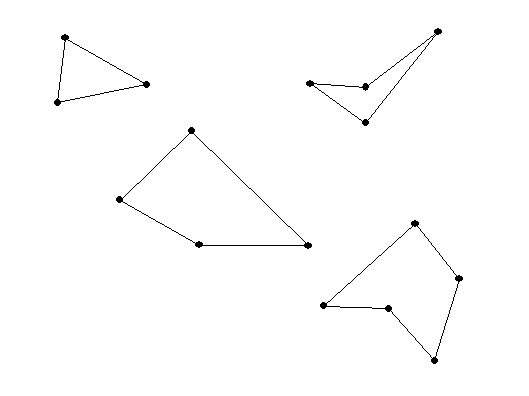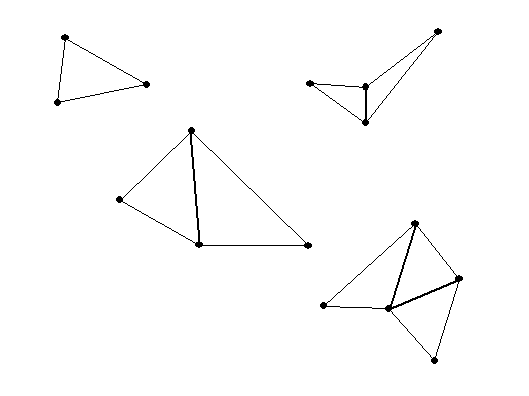# Thursday  February 2

Geometric Puzzle Foundations
• Measuring angles, lengths and areas.
• Triangles  : add to 180 degrees- straight angle [Illustrate physically and with wingeometry]
• Squares, rectangles  : 90 degree/ right angle
• parallelograms: opposite angles are congruent, sum of consecutive angles =180 degrees
• Dissections, cut and paste methods of measurement.
• Cutting and reassembling polygons.
• The "Square Me" Puzzle
• The triangle, quadrilateral, pentagon, and hexagon.

More on measurements of angles of polygons with n sides. .
When n = 3 this is a triangle, n=4, a quadrilateral, or when n= 5, a pentagon.The sum of the measures of the interior angles of a triangle is 180 degrees.
What about a quadrilateral? and a pentagon?  or an n sided polygon  ( an "n -gon")?so the sum of its interior angles is 2 * 180 = 360.
• A pentagon can be made from 3 triangles... so the sum of its interior angles is 3* 180 = 540. If the pentagon has all angles congruent( of equal measurement) then each angle will be 540/5 = 108 degrees!
• A hexagon can be made from 4 triangles... so the sum of its interior angles is 4* 180 = 720. If the hexagon has all angles congruent( of equal measurement) then each angle will be 720/6 = 120 degrees!

From the figures we saw that for a quadrilateral (n =4), which can be dissected into two triangles, the sum is 2*180= 360 degrees.
And for a pentagon (n=5) which can be dissected into 3 triangles, the sum is 3*180=540 degrees.

 # of sides # of Triangles Sum of Interior <‘s If equal, Measure of a single < 3 1 180 60 4 2 360 90 5 3 540 108 6 4 720 120 N ?

In general: the sum of the interior angles in a n sided polygon is
(n-2) *180 degrees.

A regular  polygon is a polygon where the sides are all of equal length and the angles are all congruent (or of equal measure).

Question: what is the measure of an individual angle in a regular polygon with n sides?

For a triangle, the individual angle is 180/3 = 60 degrees.
For a square, the individual angle is  360/4=90 degrees.
For a regular pentagon.... 3*180/5 = 540/5 =108  degrees.

Now for a HEXAGON (6 sides) the sum of the angles is 720 degrees.
So ... for a REGULAR HEXAGON

the individual angle is  4*180/6 =720/6 =120 degrees.

In general: The individual angle for a regular polygon with n sides is (n-2)*180/n degrees.
This can be expressed in other ways using algebra:
(n-2)*180/n = [180 n - 360] / n = 180 - 360/n.

 name of polygon degrees of the interior  measure of each angle 360 degrees divided  by # in Column 2 equilateral triangle 3 60 360 / 3 = 120 square 4 90 360/4= 90 regular pentagon 5 3*180/5= 108 360/5= 72 regular hexagon 6 4*180/6=120 360/6= 60 regular heptagon 7 5*180/7 360/7 regular octagon 8 6*180/8=135 360/8 = 45 regular dodecagon 12 10*180/12=1800/12=150 360/12=30

Cutting and reassembling polygons.

Convex:    Any two points in the figure have a line segment connecting them. If that line segment is always  inside the figure, then the figure is called "convex".

Making Dissection Puzzles [more links] :

Dissection of the plane--- Tilings of the plane:
Tessellations

• Activity: Tile plane with playing cards.
• FAPP watch Video: Possible tiles and tilings.
• One Regular Convex Polygon.
• Two or more Regular Convex Polygons.
• Other Polygon Tilings.
• Non polygonal tilings.

• One polygonal Tile: Quadrilateral Activity.
• An on-line tool for making tilings of the plane
• Regular and semiregular Tilings of the plane. Some explorations - classification.
• A tiling is a regular tiling if (i)it has a single tile shape that is a regular polygon and (ii) the vertices and edges of the tiles coincide (no overlapping edges)
• A tiling is a semi-regular tiling if (ii) each tile shape is a regular polygon, (ii) the vertices and edges of the tiles coincide (no overlapping edges) and (iii) every vertex has the same polygon types arranged around it.